# asp新手求教大神 如何做 动态新闻列表（可翻页）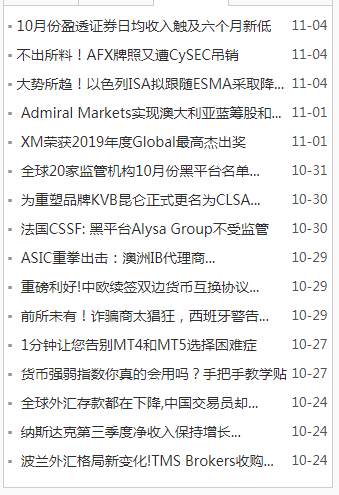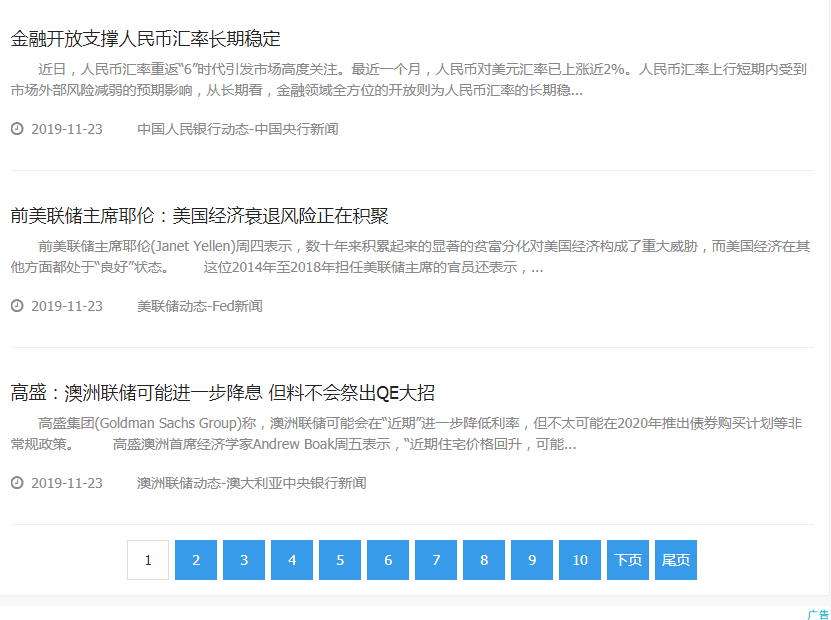http://www.waihuibang.com/centralbank/index.html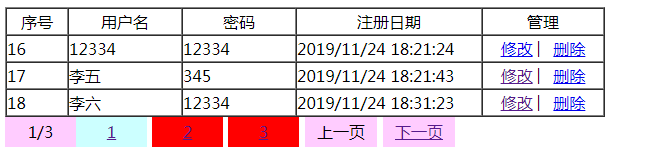2.如果以上不能实现，那要学习其它的什么课程吗？
3.是否直接用分页插件（代码） 来实现比较快？

...全文
117 点赞 收藏 4

4 条回复

if page<1 then page=1 if cint(page)>cint(rs.pageCount) then page = rs.pagecount rs.AbsolutePage = page totalrec=rs.RecordCount if totalrec mod rs.PageSize=0 then n=1 else n= totalrec \ rs.PageSize+1 end if if page-1 mod 10=0 then p=0 else p=(page-1) \ 10 end if for i=1 to rs.pagesize 你的内容 rs.movenext if rs.eof then exit for next 下面是分页 for ii=p*10+1 to (p*10+10) if cint(ii)=cint(page) then response.write "<b>"+Cstr(ii)+"</b> " else response.write "<a href='xxx.asp?Page="&Cstr(ii)&"'>"+Cstr(ii)+"</a> " end if if ii=n then exit for next

liang961 2019-11-29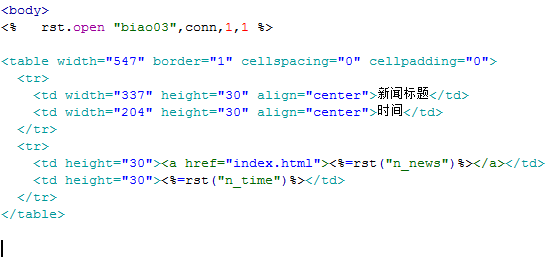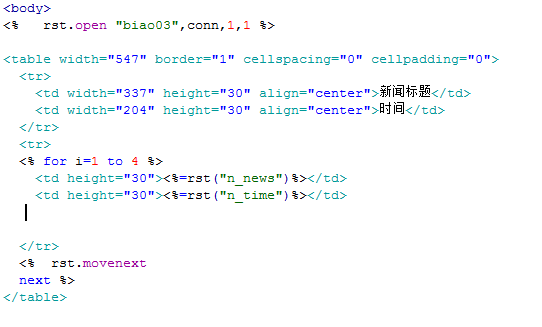never exists 2019-11-28

2.8w+

ASP即Active Server Pages，是Microsoft公司开发的服务器端脚本环境。